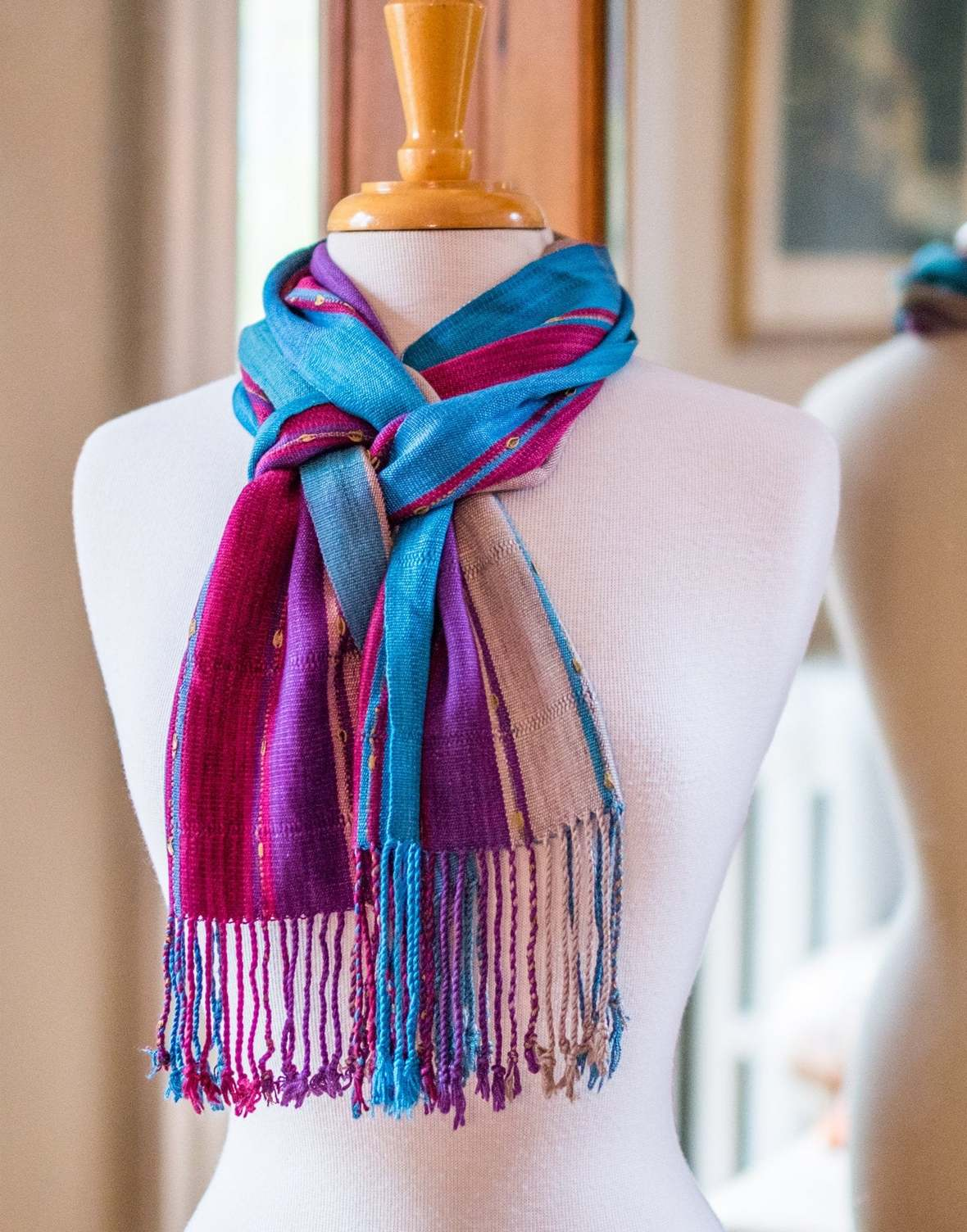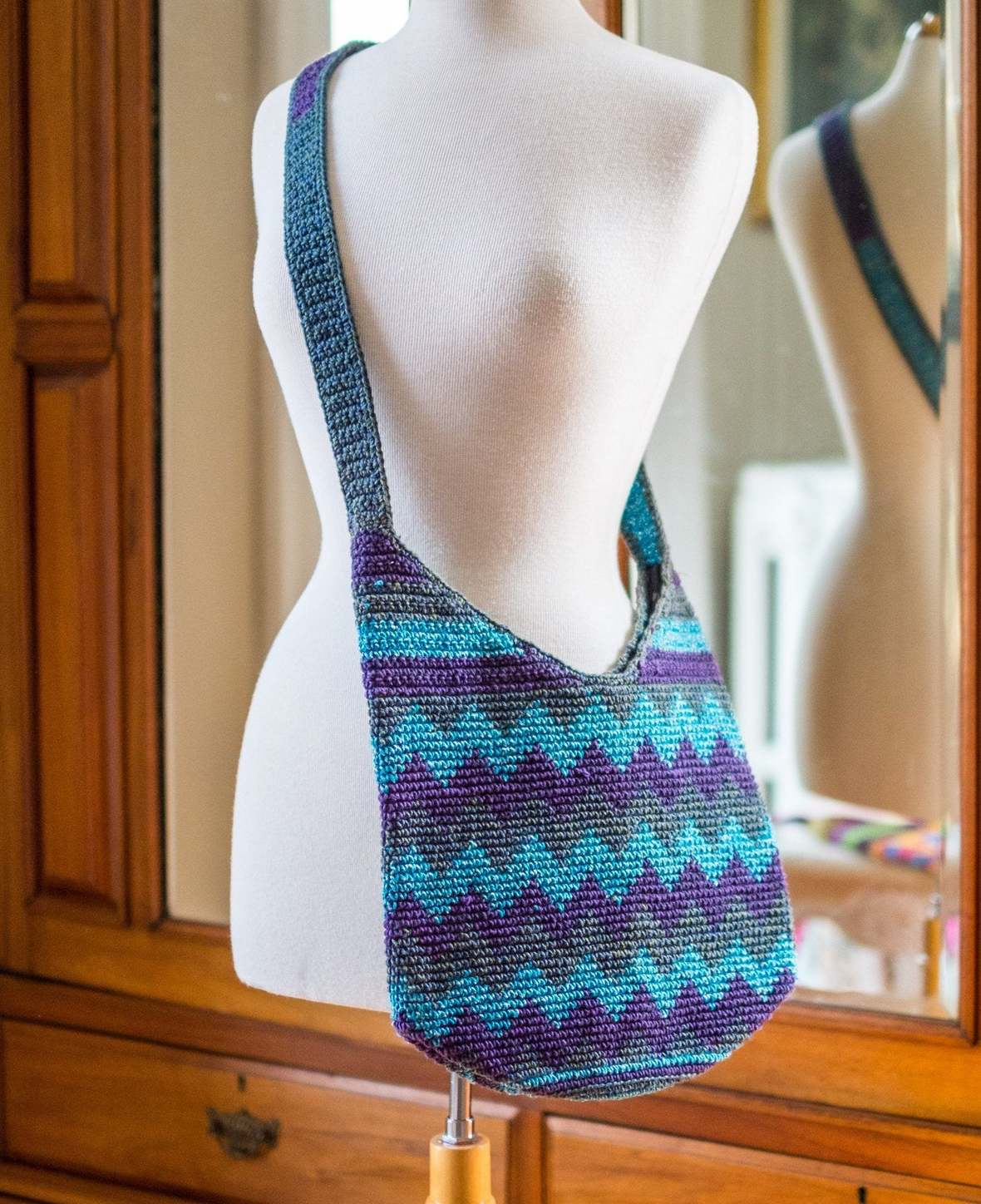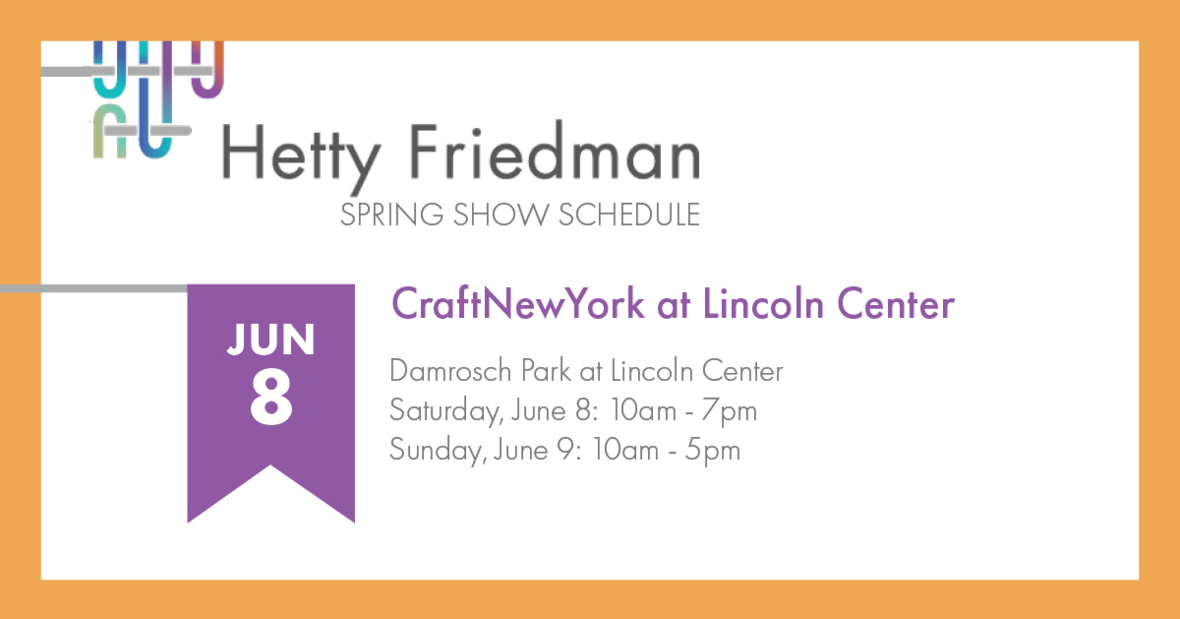Like   +1   intable div table+table div table td,table.module-1{width:100%;padding:0}table div table+table div table{width:100%;float:none;margin-left:auto;margin-right:auto;padding:0}table div table+table div table a{border:0 none;text-decoration:none}table div table+table div table img{width:100%!important;border:0 none;text-decoration:none}/* styles */
 /* styles */ Come give me a birthday hug at CraftNewYork. There will be 175 thoughtfully curated fine arts and crafts people from across the country (including me!). It's free–bring your friends and family for a fun and interesting summer outing. Damrosch Park at Lincoln Center 62nd St between Columbus & Amsterdam Avenues Booth # G704 Saturday June 8: 10am - 7pm Sunday June 9: 10am - 5pm
 table div table+table+table+table div table{width:100%;padding:0}table div table+table+table+table div table img{width:96.23%;padding:0;float:none}table div table+table+table+table div table td{width:100%;padding:0 1.88% 18px}/* styles */# A birthday gift from me to you.

## Free shipping for the month of June at my Etsy stop.

 /* styles */ Thank you for your support throughout the years. Don't forget to check out the sale section for fun favorites at a lower price!A bamboo scarf for those breezy spring days and cool summer nights.Great energy in this color combo.
 /* styles */ Like what you see? Click the photos to buy now.Guatemalan hand-crocheted bag for your next beach day! My favorite colors.A bamboo scarf for those breezy spring days and cool summer nights.

## Update your collection–see all the new things.

 table div table+table+table+table+table+table+table+table+table+table+table+table div table{width:100%;padding:0}table div table+table+table+table+table+table+table+table+table+table+table+table div table img{width:96.23%;padding:0;float:none}table div table+table+table+table+table+table+table+table+table+table+table+table div table td{width:100%;padding:0 1.88% 18px}/* styles */# It's the last show of the season!table div table+table+table+table+table+table+table+table+table+table+table+table+table+table+table div table{width:100%;padding:0}table div table+table+table+table+table+table+table+table+table+table+table+table+table+table+table div table img{width:96.23%;padding:0;float:none}table div table+table+table+table+table+table+table+table+table+table+table+table+table+table+table div table td{width:100%;padding:0 1.88% 18px}/* styles */# Don't miss a thing.

Click the buttons below to get all the latest behind-the-scenes photos, thought provoking articles, and special offers. And thank you for sharing my newsletter.

 table div table+table+table+table+table+table+table+table+table+table+table+table+table+table+table+table+table div table{width:100%;padding:0}table div table+table+table+table+table+table+table+table+table+table+table+table+table+table+table+table+table div table table{padding:0;float:left!important;width:34.716%!important}table div table+table+table+table+table+table+table+table+table+table+table+table+table+table+table+table+table div table table+table+table td,table div table+table+table+table+table+table+table+table+table+table+table+table+table+table+table+table+table div table td{padding-left:0;padding-right:0}table div table+table+table+table+table+table+table+table+table+table+table+table+table+table+table+table+table div table table td,table div table+table+table+table+table+table+table+table+table+table+table+table+table+table+table+table+table div table table+table td{padding-left:0;padding-right:20px}table div table+table+table+table+table+table+table+table+table+table+table+table+table+table+table+table+table div table table+table{float:left!important;width:34.528%!important}table div table+table+table+table+table+table+table+table+table+table+table+table+table+table+table+table+table div table table+table+table{float:left!important;width:30.756%!important}/* styles */
 table div table+table+table+table+table+table+table+table+table+table+table+table+table+table+table+table+table+table div table{width:100%;padding:0}table div table+table+table+table+table+table+table+table+table+table+table+table+table+table+table+table+table+table div table img{width:96.23%;padding:0;float:none}table div table+table+table+table+table+table+table+table+table+table+table+table+table+table+table+table+table+table div table td{width:100%;padding:0 1.88% 18px}/* styles */# Have you forgotten all the ways to wear your mobius shawl?

## Check out this video and pass it along to your friends!

 table div table+table+table+table+table+table+table+table+table+table+table+table+table+table+table+table+table+table+table+table div table{width:100%;padding:0}table div table+table+table+table+table+table+table+table+table+table+table+table+table+table+table+table+table+table+table+table div table img{width:96.23%;padding:0;float:none}table div table+table+table+table+table+table+table+table+table+table+table+table+table+table+table+table+table+table+table+table div table td{width:100%;padding:0 1.88% 18px}/* styles */## Visit my website: hettyfriedmandesigns.com

Email: hetty.friedman@gmail.com Phone: 617.512.5344